# GSEB Solutions Class 11 Maths Chapter 10 Straight Lines Miscellaneous Exercise

Gujarat Board GSEB Textbook Solutions Class 11 Maths Chapter 10 Straight Lines Miscellaneous Exercise Textbook Questions and Answers.

## Gujarat Board Textbook Solutions Class 11 Maths Chapter 10 Straight Lines Miscellaneous Exercise

Question 1.
Find the value of k for which the line (k – 3)x – (4 – k2)y + h2 – 7k + 6 = 0
(a) is parallel to x-axis
(b) is parallel to y-axis
(c) passes through the origin.
Solution:
(a) Any line parallel to x-axis is of the form y = p, i.e., coeff. of x = 0.
∴ In equation (k – 3)x – (4 – k2)y + k2 – 7k + 6 = 0,
coefficient of x = k – 3 = 0.
∴ k = 3.

(b) Any line parallel to y-axis is of the form x = q.
∴ coefficient of y = 0.
In (k – 3)x – (4 – k2)y + k2 – 7x + 6 = 0,
coefficient of y = 4 – k2 = 0.
∴ k = ±2.

(c) If the line passes through the origin, then
x = 0, y = 0 satisfy the equation.
∴ Putting x = 0, y = 0 in
(k – 3)x – (4 – k2)y + k2 – 7k + 6 = 0,
we get k2 – 7k + 6 = 0.
or (k – 6)(k – 1) = 0.
∴ k = 6, 1.Question 2.
Find the values of θ and p, if the equation xcos θ + ysin θ = p is the normal form of the line $$\sqrt{3}$$x + y + 2 = 0.
Solution:
The given line is $$\sqrt{3x}$$ + y = – 2.
Dividing by $$\sqrt{(\sqrt{3})^{2}+1^{2}}$$ = 2, we get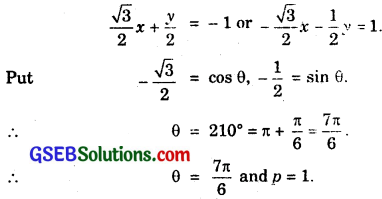Question 3.
Find the equations of the lines which cut off intercepts on the axes whose sum and product are 1 and – 6 respectively.
Solution:
Let a and b be the intercepts, the lines makes on the axes
Sum of intercepts = a+ b = 1 …………………. (1)
Product of intercepts = ab = – 6 ……………………. (2)
From (1) and (2), a(1 – a) = – 6
or a – a2 = – 6 or a2 – a – 6 = 0.
⇒ (a – 3)(a + 2) = 0
∴ a = 3, – 2
∴ b = – 2, 3
∴ Required lines are
$$\frac{x}{2}$$ + $$\frac{y}{2}$$ – 1 and $$\frac{x}{- 2}$$ + $$\frac{y}{3}$$ = 1.Question 4.
What are the points on y-axis whose distances from $$\frac{x}{3}$$ + $$\frac{y}{4}$$ = 1 are 4 units.
Solution:
Any point on y-axis is (0, y1)
∴ Perpendicular ditance of (0, y1) to $$\frac{x}{3}$$ + $$\frac{y}{4}$$ = 1
or 4x + 3y – 12 = 0 is
∴ $$\frac{0+3 y_{1}-12}{\sqrt{4^{2}+3^{2}}}$$ = ± 4 or $$\frac{3 y_{1}-12}{5}$$ = ± 4.
∴ 3y1 – 12 = ± 20.
For +ve sign, 3y1 – 12 = 20 ⇒ y1 = $$\frac{32}{3}$$.
For -ve sign, 3y1 – 12 = – 20 ⇒ y1 = $$\frac{- 8}{3}$$.
∴ The points on the y-axis are (0, $$\frac{32}{3}$$) and (0, $$\frac{- 8}{3}$$).

Question 5.
Find perpendicular distance of the line joining the points (cos θ, sin θ) and (cos ∅, sin ∅) from the origin.
Solution:
Equation of the line joining (cos θ, sin θ) and (cos ∅, sin ∅) is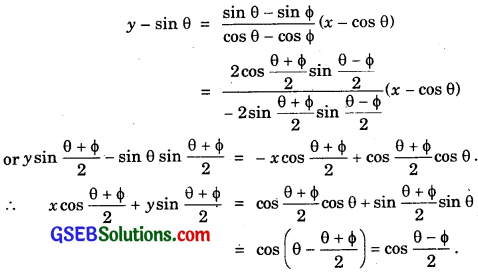∴ The line passing through (cos θ, sin θ) and (cos ∅, sin ∅) is
x cos $$\frac{θ+∅}{2}$$ + ysin $$\frac{θ+∅}{2}$$ = cos $$\frac{θ-∅}{2}$$.
∴ Perpendicular distance from the originQuestion 6.
Find the equation of the line parallel to y-axis and drawn through the point of intersection of lines x – 7y + 5 = 0 and 3x + y = 0.
Solution:
The point of intersection of the lines
x – 7y + 5 = 0 and 3x + y = 0 is obtained by solving these equations.
Putting y = – 3x in x – 7y + 5 = 0, we get
x – 7(- 3x) + 5 = 0 or x + 21x + 5 = 0.
or 22x + 5 = 0 or x = $$\frac{- 5}{22}$$.
Any line parallel to y-axis is x = x1.
Here, x1 = $$\frac{- 5}{22}$$.
∴ The equation of the line parellel toy-axis and passing through the intersection of the given lines is x = $$\frac{- 5}{22}$$ or 22x + 5 = 0.

Question 7.
Find the equation of a line drawn perpendicular to the line $$\frac{x}{4}$$ + $$\frac{y}{6}$$ = 1, through the point where it meets the y-axis.
Solution:
Putting x = 0, we get y = 6. The line $$\frac{x}{4}$$ + $$\frac{y}{6}$$ = 1 meets the y-axis.
Solution:
Putting x = 0, we get y = 6. The line $$\frac{x}{4}$$ + $$\frac{y}{6}$$ = 1 meets the y-axis at (0, 6).
The slope of the given line $$\frac{x}{4}$$ + $$\frac{y}{6}$$ = 1
or 6x + 4y = 24
is – $$\frac{6}{4}$$ = – $$\frac{3}{2}$$.
∴ Slope of line BC perpendicular to
AB = $$\frac{2}{3}$$
∴ Equation of BC is y – 6 = $$\frac{2}{3}$$(x – 0).
or 3y – 18 = 2x
or 2x – 3y + 18 = 0.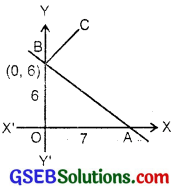Question 8.
Find the area of traingle formed by the lines y – x = 0, x + y = 0 and x – k = 0.
Solution:
The given lines are
y – x = 0 ………………….. (1)
x + y = 0 ………………….. (2)
x – k = 0 ………………….. (3)
From (1) and (2), x = 0, y = 0.
From (2) and (3), x = k, k + y = 0 or y = – k.
From (3) and (1), x = k, y – k = 0 or y = k.
∴ The vertices of the traiangle are O(0, 0), B(k, – k) and A(k, k).
∴ Base of the ∆ OAB = 2k and perpendicular distance from O to AB = k.
∴ Area of ∆ OAB = $$\frac{1}{2}$$ × h × 2k.
= k2 sq.units.Question 9.
Find the value of p so that the three lines 3x + y – 2 = 0, px + 2y – 3 = 0 and 2x – y – 3 = 0 may intersect at one point.
Solution:
Consider the lines
3x + y = 2 ……………………. (1)
2x – y = 3 ………………….. (2)
Adding 5x = 5 or x = 1.
Putting x = 1 in (1), 3 + y = 2.
∴ y = – 1.
∴ Lines (1) and (2) intersect at (1, – 1).
This point will lie on the line px + 2y – 3 = 0. if
p × 1 + 2(- 1) – 3 = 0 or p = 5.
∴ For p = 5, the given lines intersect at (1, – 1).Question 10.
If three lines whose equations are y = m1x + c1, y = m2x + c2 and y = m3x + c3 are concurrent, then show that m1(c2 – c3) + m2(c3 – c1) + m3(c1 – c2) = 0.
Solution:
Consider the lines
y = m1x + c1 ………………….. (1)
and y = m2x + c2 ………………… (2)
Subtracting, we get
0 = (m1 – m2)x + c1 – c2.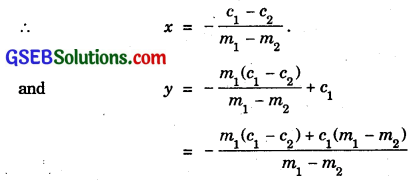Putting the values of x and y in the third equation
y = m3x + c3, we get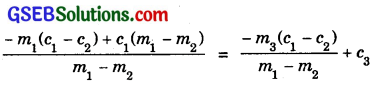⇒ – m1(c1 – c2) + c1(m1 – m2) + m3(c1 – c2) – c3(m1 – m2) = 0
⇒ m1(- c1 + c2 + c1 – c3) + m2(- c1 + c3) + m3(c1 – c2) = 0
i.e; m1(c2 – c3) + m2(c3 – c1) + m3(c1 – c2) = 0.

Question 11.
Find the equation of the lines passing through the points (3, 2) and making an angle of 45° with the line x – 2y = 3.
Solution:
Le the line AB be x – 2y = 3.
Its slope = $$\frac{1}{2}$$.
Let m be the slope of the line PA passing through the point P(3, 2).
Angle between PA and AB is 45°.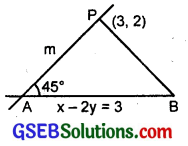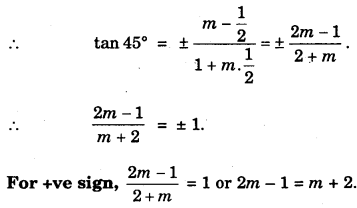or m = 3.
Equation of the line PA, passing through P(3, 2) and making an angle of 45° with AB is
y – 2 = 3(x – 3) = 3x – 9
or 3x – y – 9 + 2 = 0 or 3x – 7 = 7.
For – ve sign,
$$\frac{2m-1}{m+2}$$ = – 1 or 2m – 1 = – m – 2.
or 3m = – 1 ⇒ m = – $$\frac{1}{3}$$.
∴ Equation of PB is
∴ y – 2 = – $$\frac{1}{3}$$(x – 3)
3y – 6 = – x + 3 or x + 3y – 6 – 3 = 0
or x + 3y – 9 = 0.Question 12.
Find the equation of the line passing through the point of intersection of the lines 4x + 7y – 3 = 0 and 2x – 3y + 1 = 0 and that has equal intercepts on the axis?
Solution:
The given lines are
2x – 3y = – 1 ……………… (1)
4x + 7y = 3 ……………. (2)
Multiplying eq. (1) by 2, we get
4x – 6y = – 2 ………………. (3)
Substracting (3) from (2), we get
13y = 5.
∴ y = $$\frac{5}{13}$$.
Putting the value of y in (1), 2x – $$\frac{3×5}{13}$$ = – 1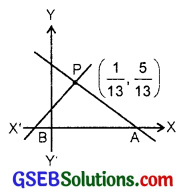Let this point be denoted by P.
PA and PB are the lines that make equal intercepts on the axes.
They make angles of 135° and 45° with positive direction of x-axis.
∴ Their slopes are tan 135° and tan 45° i.e., – 1 and 1 respectively.
∴ Equation of PA is
y – $$\frac{5}{13}$$ = – (x – $$\frac{1}{13}$$)
or 13y – 5 = – 13x + 1
13x + 13y – 6 = 0.
Equation of PB is
y – $$\frac{5}{13}$$ = 1. (x – $$\frac{1}{13}$$).
or 13y – 5 = 13x – 1
or 13x – 13y + 5 – 1 = 0
or 13x – 13y + 4 = 0.

Question 13.
Show that the equation of the line passing through the origin and making an angle θ with the line y = mx + c is $$\frac{y}{x}$$ = $$\frac{m \pm \tan \theta}{1 \mp m \tan \theta}$$.
Solution:
Let PQ be the line y = mx + c, whose slope is in.
The line PO makes an angle θ with PQ. Let its slope be m1.∴ Equation of the line OP is
y = m1x.
or y = $$\frac{y}{x}$$ = m
∴ $$\frac{y}{x}$$ = $$\frac{m \pm \tan \theta}{1 \mp m \tan \theta}$$.Question 14.
In what ratio, the line segment joining (- 1, 1) and (5, 7) is divided by the line x + y = 4?
Solution:
The line segment joining the points A(- 1, 1) and B(5, 7) is divided by P(x1, y1) in some ratio. Let this ratio be k : 1.
∴ Point P is ($$\frac{5k-1}{k+1}$$, $$\frac{7k+1}{k+1}$$).
The point P lies on the line x + y = 4.
∴ $$\frac{5k-1}{k+1}$$ + $$\frac{7k+1}{k+1}$$ = 4.
or 5k – 1 + 7k + 1 = 4k = 4.
or 8k = 4.
∴ k = $$\frac{1}{2}$$.
∴ P divides AB in the ratio $$\frac{1}{2}$$ : 1, i.e; 1 : 2.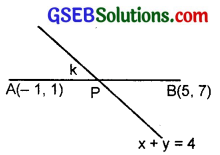Question 15.
Find the distance of the line 4x + 7y + 5 = 0 from the point (1, 2) along the line 2x – y = 0.
Solution:
The equation of line AB is 4x + 7y + 5 = 0 ………………… (1)
The equation of PQ is 2x – y = 0 ………………… (2)
The point P is (1, 2).
From eq. (2) is y = 2x.
Putting it in eq. (1), we get
4x + 7 × 2x + 5 = 0.
⇒ 18x + 5 = 0 ⇒ x = $$\frac{- 5}{18}$$.∴ y = 2x = – 2 × $$\frac{5}{18}$$ = – $$\frac{5}{9}$$.
∴ The point Q, where the given lines meet is ($$\frac{- 5}{18}$$, $$\frac{- 5}{9}$$).
The point P is (1, 2).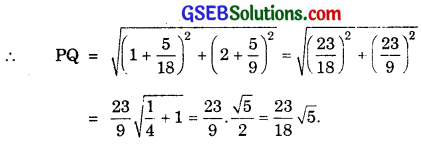Question 16.
Find the direction in which a straight line must be drawn through the point (- 1, 2) so that its point of intersection with the line x + y = 4 may be at a distance of 3 units from this point.
Solution:
A line passing through P(- 1, 2) is
y – 2 = m(x + 1),
where m is its slope.
⇒ y = mx + m + 2
Putting this value of y in x + y = 4, we get
x + mx + m + 2 = 4.
or (1 + m)x = 4 – 2 – m
∴ x = $$\frac{2-m}{1+m}$$.∴ The co-ordinates of Q the point of intersection Q of AB and PQ is ($$\frac{2-m}{1+m}$$, $$\frac{2+5m}{1+m}$$).
The point P is (- 1, 2) and PQ = 3 (Given).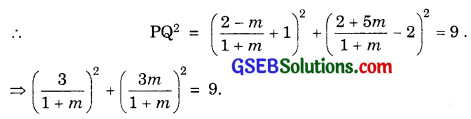⇒ 1 + m2 = (1 + m2)2 = 1 + 2m + m2
⇒ m = 0.
∴ Slope of PQ is zero i.e; it is parallel to x-axis.Question 17.
The hypotenuse of a right traiangle has its ends at the points (1, 3) and (- 4, 1). Find the equation of the legs (perpendicular sides) of the triangle.
Solution:
Let ABC be the right triangle such that ∠C = 90°,
There are infinitely many such lines.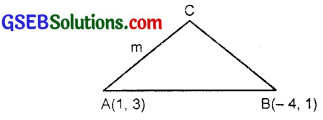Let m be the slope of AC. Then, the slope of BC = – $$\frac{1}{m}$$.
∴ Equation of AC is y – 3 = m(x – 1).
or x – 1 = $$\frac{1}{m}$$(y – 3).
Equation of BC is x + 4 = – m(y – 1).
⇒ y – 1 = – $$\frac{1}{m}$$(x + 4).
For a given value of m, we can find these equations.
For m = 0, these lines are x + 4 = 0, y – 3 = 0.
For m = ∞, the lines are x – 1 = 0, y – 1 = 0.

Question 18.
Find the image of the point (3, 8) with respect to a line x + 3y = 7, assuming the line to be a plane mirror.
Solution:
Let AB be the line x + 3y = 7 and let the image of P(3, 8) be Q(x1, y1).
Middle point M($$\frac{x_{1}+3}{2}, \frac{y_{1}+8}{2}$$) lies on AB.
∴ ($$\frac{x_{1}+3}{2}$$) + 3($$\frac{y_{1}+8}{2}$$) = 7
or x1 + 3 + 3y1 + 24 = 14
or x1 + 3y1 + 13 = 0 ………………… (1)
Now, slope of AB = – $$\frac{1}{3}$$or y1 – 8 = 3(x1 – 3) = 3x1 – 9
or y1 = 3x1 – 1 ………………………. (2)
Putting the value of y1 in (1), we get
x1 + 3(3x1 – 1) + 13 = 0
10x1 + 10 = 0 or x1 = – 1
Putting x1 = – 1 in eq. (2), we get
y1 = – 3 – 1 = – 4.
∴ The image Q of P is (- 1, – 4).Question 19.
1f the line y = 3x + 1 and 2y = x +3 are equally inclined to the line y = mx + 4, find the value of m.
Solution:
Slope of the line PQ, i.e., y = 3x + 4 is 3.
Slope of QR = m.
The angle θ between the lines is given by
tan θ = |$$\frac{m-3}{1+3m}$$|.
Slope of the line PR, i.e.,
2y = x + 3 is $$\frac{1}{2}$$.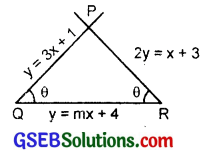Slope of QR is m.
The angle between PR and QR is given by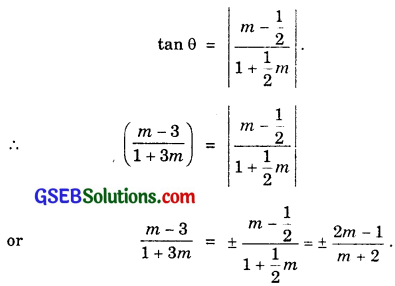For +ve sign, $$\frac{m-3}{3m+1}$$ = $$\frac{2m-1}{m+2}$$.
or (3m + 1)(2m – 1) = (m – 3)(m + 2)
or 6m2 – m – 1 = m2 – m – 6
5m2 = – 5 or m2 = – 1 (Not admissible)
For -ve sign, $$\frac{m-3}{3m+1}$$ = – $$\frac{2m-1}{m+2}$$.
or (3m + 1)(2m – 1) = – (m – 3)(m + 2).
or 6m2 – m – 1 = – m2 + m + 6.
or 7m2 – 2m – 7 = 0.Question 20.
If the sum of perpendicular distances of a variable point P(x, y) from the lines x + y – 5 = 0 and – 2y + 7 = 0 is always 10, show that P must move on a line.
Solution:
Perpendicular distance p1 from P(x, y) to the line x + y – 5 = 0 is given by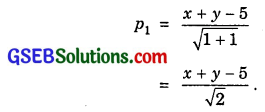Perpendicular distance p2 from P(x, y) to the line 3x – 2y + 7 = 0 is given bywhich is the equation of a straight line. Hence, P moves on a line.Question 21.
Find the equation of the line, which is equidistant from parallel lines 9x + 6y – 7 = 0 and 3x + 2y + 6 = 0.
Solution:
The given parallel lines are
9x + 6y – 7 = 0 …………….. (1)
and 3x + 2y + 6 = 0
Multiplying by 3, we get
9x + 6y + 18 = 0 …………………. (2)
Let the third line parallel to the lines (1) and (2) and then which is equidistant from them be
9x + 6y + c = 0 ………………… (3)
Distance between (1) and (3) = $$\frac{|-7-c|}{\sqrt{9^{2}+6^{2}}}$$.
Distance between (2) and (3) = \frac{|c-18|}{\sqrt{9^{2}+6^{2}}}.
∴ The third line being equidistant from the given two lines, we get
$$\frac{|7+c|}{\sqrt{117}}$$ = $$\frac{|c-18|}{\sqrt{117}}$$
or 7 + c = -(c – 18) or 2c = 11.
∴ c = $$\frac{11}{2}$$.
∴ The line mid-way between the given lines is
9x + 6y + $$\frac{11}{2}$$ = 0 or 18x + 12y + 11 = 0.Question 22.
A ray of light passes through the point (1, 2) reflects on the x-axis at a point A and the reflected ray passes through the point (5, 3). Find the co-ordinates of A.
Solution:
Angle of incidence = Angle of reflection.
If AN ⊥ OX, then
∠PAN = ∠QAN,
where P is (1, 2) and Q is (5, 3).
⇒ ∠QAX = ∠PAO.
∴ If ∠QAX = θ, then ∠PAO = θ.
∴ ∠XAP = 180° – θ.
∴ Slope of AQ = tan θ = $$\frac{3-0}{5-k}$$.
where the point A is (k, 0).
Slope of AP = tan(180° – θ) = $$\frac{2-0}{1-k}$$ = – tan θ.
∴ $$\frac{3}{5-k}$$ = – $$\frac{2}{1-k}$$
or 3 – 3k = – 10 + 2k
or 5k = 13.
∴ k = $$\frac{13}{5}$$.∴ The point A is ($$\frac{13}{5}$$, 0).

Question 23.
Prove that the product of the lengths of perpendiculars drawn from the point ($$\sqrt{a^{2}-b^{2}}$$, 0) and (- $$\sqrt{a^{2}-b^{2}}$$, 0) to the line $$\frac{x}{a}$$ cos θ + $$\frac{y}{b}$$ sin θ = 1 is b2.
Solution:
The perpendicular distances p1 from ($$\sqrt{a^{2}-b^{2}}$$, 0) and (- $$\sqrt{a^{2}-b^{2}}$$, 0) to the line cos θ + sin θ = 1 areQuestion 24.
A person standing at the junction (crossing) of two straight paths represented by the equations 2x – 3y = 4 and 3x + 4y – 5 = 0 wants to reach the path whose equation is 6x – 7y + 8 = 0 in the least time. Find the equation of the path that he should follow.
Solution:
Equation of the two paths OA and OB are
2x – 3y – 4 = 0 ……………… (1)
3x + 4y – 5 = 0 ……………… (2)
Solving these equations, we get
$$\frac{x}{15+16}$$ = $$\frac{y}{-12+10}$$ = $$\frac{1}{8+9}$$
∴ x = $$\frac{31}{17}$$, y = $$\frac{- 2}{17}$$.
∴ These paths meet at ($$\frac{31}{17}$$, $$\frac{- 2}{17}$$).The third path is AB whose equation is 6x – 7y + 8 = 0.
The shortest path from O to the path AB is the perpendicular path from O to AB.
∴ Slope of AB = $$\frac{6}{7}$$
∴ Slope of ⊥ path = $$\frac{-7}{6}$$
∴ Equation of perpendicular path OM is
y + $$\frac{2}{17}$$ = – $$\frac{7}{6}$$(x – $$\frac{31}{17}$$)
⇒ y + $$\frac{2}{17}$$ = – $$\frac{7}{6}$$x + $$\frac{217}{102}$$.
Multiplying by 102, we get
102y + 12 = – 119x+ 217
or 119x + 102y – 205 = 0.
∴ Equation of the shortest path is
119x + 102y – 205 = 0.
Hence, the time taken to reach AB will be minimum.